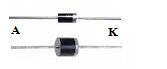## Saturday, November 14, 2009

### Menguji Dioda Menggunakan Multi Meter

Menguji Dioda Menggunakan Multi Meter

In the diode consists of cathode and anode, and this is given one mark. Then the steps using a multimeter diode test is as follows:
1. Turn the switch on the Ohm, let R x 1K
2. Investigators Attach the red to the cathode (+) and black Investigators at the anode (-) diode
3. If the needle moves multimeter that is good, but if the needle does not move means diodes have been broken or damaged
4. Then a black investigator then attached to the cathode (+) and red pencolok attached to the anode (-). When the needle multimeter silent, means the diode is in good condition but if the move means that the diode has been leaked (damaged)

About Diode

A diode is the basic electronic component. It’s property is that it can conduct only in one direction. A diode can be made by joining a p type semiconductor and an n type semiconductor. At the junction point there will be a voltage drop due to the migration of carriers. The voltage at this barrier is known as barrier potential. Ideally the barrier potential is zero. But in practical case there will be a drop of 0.7 Volts.When the P side of diode is connected to the positive of power supply and N region is connected to the negative of supply, the diode is said to be in forward biased condition. At this condition, if the applied voltage is greater than the barrier potential of the diode, it starts conduction. After the diode is arrived in the conduction mode, the drop across it remains at 0.7 V. After the conduction starts, if the voltage is increased further, current trough it increases linearly with voltage.

When the supply voltage is reversed the diode is said to be in reverse biased condition. Here there is no conduction at lower voltage values .If we increase the voltage value further ,it is observed that at a voltage the current sharply increases due to the breakdown of the P-N junction. This damages the device.

Skema Rangkaian Elektronika